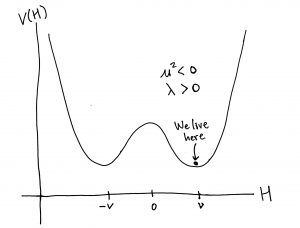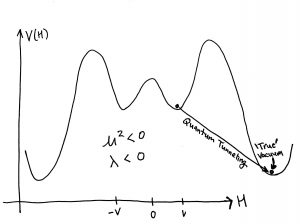# The end of the Universe?

Hello nibblers,

Don’t worry, my title doesn’t refer to the recent US election. Only to the end of the Universe due to the Higgs boson. Let me explain…

Last time I wrote, I discussed new methods for determining whether the Higgs boson interacts with itself. As you will recall, establishing whether this is the case is crucial for determining the precise form of the Higgs scalar potential which is responsible for breaking electroweak symmetry and generating masses for the W and Z bosons via the Higgs mechanism. You might also remember that I briefly mentioned that these Higgs self interactions might have deep implications for the stability of our universe. Since I think this topic is interesting enough for its own post I wanted to go into a little more. Its a fascinating topic, but the details can get hairy pretty quickly so ill keep it as simple as possible and just try and give a flavor before going into more detail in future posts.

In the Standard Model, the Higgs potential determines the ‘vacuum’ of our universe, which by definition is the lowest energy state and presumably the one in which we live in today. We can think of the Higgs potential as a ‘background, along with spacetime, in which the various processes which occur in the Universe are acted out. In the Standard Model the Higgs potential before electroweak symmetry can be written as a very simple polynomial in$H$ (after requiring gauge invariance and renormalizability),$V(H) = \mu^2 H^2 + \lambda H^4$.

Assuming$\mu^2 > 0$ and$\lambda > 0$, this looks like the function drawn in Figure 1. Visually it is easy to see that$H = 0$ is a minimum of$V(H)$. We call this a stable minimum because if you imagine a ball sitting at the bottom of the ‘bowl’ and moving it to the left or right it will simply role back down to$H = 0$. Since at this point the energy is a minimum, we call it the vacuum. In order for our universe to be stable we must live in a minimum such as this, i.e. a stable vacuum.Figure 1: The Higgs potential for and . This potential has a stable minimum at .

If on the other hand we have$\mu^2 > 0$ and$\lambda < 0$, the potential now looks like that in Figure 2. Clearly now$H=0$ is no longer a stable point as can be understood by again considering a ball sitting at$H = 0$ and moving it to the left or right. As we see in Figure 2, instead of rolling back to$H = 0$ as before, it will simply keep rolling down to the left or right to arbitrarily large values of$H$, never to return to$H = 0$. We say this potential is unstable and something like it would be disastrous for our universe. So we see in this very simple example already how the sign of$\lambda$ is crucial for determining whether the vacuum, and as a consequence our universe, is stable.

Once electroweak symmetry breaking occurs the potential becomes a little more complicated, but more interesting. Still, the stability of our vacuum will depend on the sign of$\lambda$. We can see this by considering the case$\mu^2 < 0$ and$\lambda > 0$ for which the Higgs develops a vacuum expectation value (VEV) and breaks electroweak symmetry. The Higgs potential looks like what we see in Figure 3. Now the minimum is not at$H = 0$, but at$H = \pm v$ where$v$ is the value of the Higgs’ VEV and we can think of the two minima as equivalent due to symmetry.Figure 3: The Higgs potential for and . This potential breaks electroweak symmetry and has a stable minimum at .

The subtlety comes when we consider what happens at very large$H$. In Figure 3 the potential appears to increase forever as$H$ gets larger and thus$H = v$ would an absolute minimum of$V(H)$. But is it possible that at large enough values of$H$ we’ll find another even deeper minimum which would thus be the `true’ vacuum? This is possible if$\lambda < 0$ which could generate a potential of the form shown in Figure 4.Figure 4: The Higgs potential for and . This potential breaks electroweak symmetry and has a ‘false’ vacuum at with the ‘true’ deeper vacuum at much larger .

In this case there can be a deeper ‘well’ than the one where we currently live near$H = v$. Naively we would need enough energy to get over the large humps and fall to the deeper minimum. Thus there is no worry that our Universe will suddenly decay away to some other lowest energy state which probably would not include people and we can rest easy….buuut…this is only what would happen in a classical world.

Here the magic of quantum mechanics (or should I say terror?) comes into play. Due to quantum fluctuations even though the Higgs lives the vast majority of the time in the minimum around$H = v$ there is a tiny probability that the Higgs boson can suddenly fluctuate to very large values of$H$. If there is a deeper minimum at these large values as shown in Figure 4, then we could quantum mechanically ‘tunnel’ from our current vacuum, to the deeper ‘true’ vacuum.

Needless to say this sounds bad. So what happens in the Standard Model, is the Universe stable? The answer turns out to be closely related to the Higgs boson and top quark masses and takes us into the deep waters of renormalization, but i’ll save this interesting connection to a future post. For now we can just appreciate the importance of determining$\lambda$ by measuring the Higgs self interactions at the LHC or future colliders.

1. If you want to start learning about the conept of a vacuum here is a good place to start.
2. Here again is a basic introduction to the physics of the Standard Model Higgs boson.
The following two tabs change content below.#### Roberto Vega-Morales

Roberto Vega-Morales is currently a post-doctoral researcher in high energy theory at the University of Granada in Spain. Previously he was at the Laboratoire de Physique Thèorique in Paris France. He conducted his Ph.D studies at Northwestern University as well as Fermilab and was awarded the 2014 J.J. and Noriko Sakurai Dissertation Award in Theoretical Particle Physics. His research focuses on the phenomenology of the Higgs boson at the LHC as well as models of Supersymmetry and extended Higgs sectors. He struggled mightily with French and is happy to be speaking Spanish nowadays.#### Latest posts by Roberto Vega-Morales (see all)

CategoriesUncategorized